# A closer look at orbit integration¶

## Orbit initialization¶

### Standard initialization¶

Orbits can be initialized in various coordinate frames. The simplest initialization gives the initial conditions directly in the Galactocentric cylindrical coordinate frame (or in the rectangular coordinate frame in one dimension). Orbit() automatically figures out the dimensionality of the space from the initial conditions in this case. In three dimensions initial conditions are given either as [R,vR,vT,z,vz,phi] or one can choose not to specify the azimuth of the orbit and initialize with [R,vR,vT,z,vz]. Since potentials in galpy are easily initialized to have a circular velocity of one at a radius equal to one, initial coordinates are best given as a fraction of the radius at which one specifies the circular velocity, and initial velocities are best expressed as fractions of this circular velocity. For example,

>>> from galpy.orbit import Orbit
>>> o= Orbit([1.,0.1,1.1,0.,0.1,0.])


initializes a fully three-dimensional orbit, while

>>> o= Orbit([1.,0.1,1.1,0.,0.1])


initializes an orbit in which the azimuth is not tracked, as might be useful for axisymmetric potentials.

In two dimensions, we can similarly specify fully two-dimensional orbits o=Orbit([R,vR,vT,phi]) or choose not to track the azimuth and initialize with o= Orbit([R,vR,vT]).

In one dimension we simply initialize with o= Orbit([x,vx]).

### Initialization with physical units¶

Orbits are normally used in galpy’s natural coordinates. When Orbits are initialized using a distance scale ro= and a velocity scale vo=, then many Orbit methods return quantities in physical coordinates. Specifically, physical distance and velocity scales are specified as

>>> op= Orbit([1.,0.1,1.1,0.,0.1,0.],ro=8.,vo=220.)


All output quantities will then be automatically be specified in physical units: kpc for positions, km/s for velocities, (km/s)^2 for energies and the Jacobi integral, km/s kpc for the angular momentum o.L() and actions, 1/Gyr for frequencies, and Gyr for times and periods. See below for examples of this.

The actual initial condition can also be specified in physical units. For example, the Orbit above can be initialized as

>>> from astropy import units
>>> op= Orbit([8.*units.kpc,22.*units.km/units.s,242*units.km/units.s,0.*units.kpc,22.*units.km/units.s,0.*units.deg])


In this case, it is unnecessary to specify the ro= and vo= scales; when they are not specified, ro and vo are set to the default values from the configuration file. However, if they are specified, then those values rather than the ones from the configuration file are used.

Tip

If you do input and output in physical units, the internal unit conversion specified by ro= and vo= does not matter!

Inputs to any Orbit method can also be specified with units as an astropy Quantity. galpy’s natural units are still used under the hood, as explained in the section on physical units in galpy. For example, integration times can be specified in Gyr if you want to integrate for a specific time period.

If for any output you do not want the output in physical units, you can specify this by supplying the keyword argument use_physical=False.

### Initialization from observed coordinates or astropy SkyCoord¶

For orbit integration and characterization of observed stars or clusters, initial conditions can also be specified directly as observed quantities when radec=True is set (see further down in this section on how to use an astropy SkyCoord instead). In this case a full three-dimensional orbit is initialized as o= Orbit([RA,Dec,distance,pmRA,pmDec,Vlos],radec=True) where RA and Dec are expressed in degrees, the distance is expressed in kpc, proper motions are expressed in mas/yr (pmra = pmra’ * cos[Dec] ), and Vlos is the heliocentric line-of-sight velocity given in km/s. The observed epoch is currently assumed to be J2000.00. These observed coordinates are translated to the Galactocentric cylindrical coordinate frame by assuming a Solar motion that can be specified as either solarmotion='hogg' (2005ApJ…629..268H), solarmotion='dehnen' (1998MNRAS.298..387D) or solarmotion='schoenrich' (default; 2010MNRAS.403.1829S). A circular velocity can be specified as vo=220 in km/s and a value for the distance between the Galactic center and the Sun can be given as ro=8.0 in kpc (e.g., 2012ApJ…759..131B). While the inputs are given in physical units, the orbit is initialized assuming a circular velocity of one at the distance of the Sun (that is, the orbit’s position and velocity is scaled to galpy’s natural units after converting to the Galactocentric coordinate frame, using the specified ro= and vo=). The parameters of the coordinate transformations are stored internally, such that they are automatically used for relevant outputs (for example, when the RA of an orbit is requested). An example of all of this is:

>>> o= Orbit([20.,30.,2.,-10.,20.,50.],radec=True,ro=8.,vo=220.)


However, the internally stored position/velocity vector is

>>> print(o.vxvv)
# [1.1480792664061401, 0.1994859759019009, 1.8306295160508093, -0.13064400474040533, 0.58167185623715167, 0.14066246212987227]


and is therefore in natural units.

Tip

Initialization using observed coordinates can also use units. So, for example, proper motions can be specified as 2*units.mas/units.yr.

Similarly, one can also initialize orbits from Galactic coordinates using o= Orbit([glon,glat,distance,pmll,pmbb,Vlos],lb=True), where glon and glat are Galactic longitude and latitude expressed in degrees, and the proper motions are again given in mas/yr ((pmll = pmll’ * cos[glat] ):

>>> o= Orbit([20.,30.,2.,-10.,20.,50.],lb=True,ro=8.,vo=220.)
>>> print(o.vxvv)
# [0.79959714332811838, 0.073287283885367677, 0.5286278286083651, 0.12748861331872263, 0.89074407199364924, 0.0927414387396788]


When radec=True or lb=True is set, velocities can also be specified in Galactic coordinates if UVW=True is set. The input is then [RA,Dec,distance,U,V,W], where the velocities are expressed in km/s. U is, as usual, defined as -vR (minus vR).

Finally, orbits can also be initialized using an astropy.coordinates.SkyCoord object. For example, the (ra,dec) example from above can also be initialized as:

>>> from astropy.coordinates import SkyCoord
>>> import astropy.units as u
>>> c= SkyCoord(ra=20.*u.deg,dec=30.*u.deg,distance=2.*u.kpc,
pm_ra_cosdec=-10.*u.mas/u.yr,pm_dec=20.*u.mas/u.yr,
>>> o= Orbit(c)


In this case, you can still specify the properties of the transformation to Galactocentric coordinates using the standard ro, vo, zo, and solarmotion keywords, or you can use the SkyCoord Galactocentric frame specification and these are propagated to the Orbit instance. For example,

>>> from astropy.coordinates import CartesianDifferential
>>> c= SkyCoord(ra=20.*u.deg,dec=30.*u.deg,distance=2.*u.kpc,
pm_ra_cosdec=-10.*u.mas/u.yr,pm_dec=20.*u.mas/u.yr,
galcen_distance=8.*u.kpc,z_sun=15.*u.pc,
galcen_v_sun=CartesianDifferential([10.0,235.,7.]*u.km/u.s))
>>> o= Orbit(c)


A subtlety here is that the galcen_distance and ro keywords are not interchangeable, because the former is the distance between the Sun and the Galactic center and ro is the projection of this distance onto the Galactic midplane. Another subtlety is that the astropy Galactocentric frame is a right-handed frame, while galpy normally uses a left-handed frame, so the sign of the x component of galcen_v_sun is the opposite of what it would be in solarmotion. Because the Galactocentric frame in astropy does not specify the circular velocity, but only the Sun’s velocity, you still need to specify vo to use a non-default circular velocity.

When orbits are initialized using radec=True, lb=True, or using a SkyCoord physical scales ro= and vo= are automatically specified (because they have defaults of ro=8 and vo=220). Therefore, all output quantities will be specified in physical units (see above). If you do want to get outputs in galpy’s natural coordinates, you can turn this behavior off by doing

>>> o.turn_physical_off()


All outputs will then be specified in galpy’s natural coordinates.

### Initializing multiple objects at once¶

In all of the examples above, the Orbit instance corresponds to a single object, but Orbit instances can also contain and analyze multiple objects at once. This makes handling Orbit instances highly convenient and also allows for efficient handling of multiple objects. Many of the most computationally-intense methods have been parallelized (orbit integration; analytic eccentricity, zmax, etc. calculation; action-angle calculations) and some other methods switch to more efficient algorithms for larger numbers of objects (e.g., rguiding).

All of the methods for initializing Orbit instances above work for multiple objects. Specifically, the initial conditions can be:

• Array of arbitrary shape (shape,phasedim); needs to be in internal units (for Quantity input; see ‘list’ option below or use a SkyCoord):
• in Galactocentric cylindrical coordinates with phase-space coordinates arranged as [R,vR,vT(,z,vz,phi)];
• [ra,dec,d,mu_ra, mu_dec,vlos] or [l,b,d,mu_l, mu_b, vlos] in [deg,deg,kpc,mas/yr,mas/yr,km/s], or [ra,dec,d,U,V,W] or [l,b,d,U,V,W] in [deg,deg,kpc,km/s,km/s,kms] (ICRS where relevant; mu_ra = mu_ra * cos dec and mu_l = mu_l * cos ); use the radec=, lb=, and UVW= keywords as before
• astropy (>v3.0) SkyCoord with arbitrary shape, including velocities;
• lists of initial conditions, entries can be
• individual Orbit instances (of single objects)
• Quantity arrays arranged as in the first bullet above (so things like [R,vR,vT,z,vz,phi], where R, vR, … can be arbitrary shape Quantity arrays)
• list of Quantities (so things like [R1,vR1,..,], where R1, vR1, … are scalar Quantities
• None: assumed to be the Sun; if None occurs in a list it is assumed to be the Sun and all other items in the list are assumed to be [ra,dec,…]; cannot be combined with Quantity lists
• lists of scalar phase-space coordinates arranged as in the first bullet above (so things like [R,vR,…] where R,vR are scalars in internal units

Tip

For multiple object initialization using an array or SkyCoord, arbitrary input shapes are supported.

An example initialization with an array is:

>>> vxvvs= numpy.array([[1.,0.1,1.,0.1,-0.2,1.5],[0.1,0.3,1.1,-0.3,0.4,2.]])
>>> orbits= Orbit(vxvvs)
>>> print(orbits.R())
# [ 1.   0.1]


and with a SkyCoord:

>>> numpy.random.seed(1)
>>> nrand= 30
>>> ras= numpy.random.uniform(size=nrand)*360.*u.deg
>>> decs= 90.*(2.*numpy.random.uniform(size=nrand)-1.)*u.deg
>>> dists= numpy.random.uniform(size=nrand)*10.*u.kpc
>>> pmras= 20.*(2.*numpy.random.uniform(size=nrand)-1.)*20.*u.mas/u.yr
>>> pmdecs= 20.*(2.*numpy.random.uniform(size=nrand)-1.)*20.*u.mas/u.yr
>>> vloss= 200.*(2.*numpy.random.uniform(size=nrand)-1.)*u.km/u.s
# Without any custom coordinate-transformation parameters
>>> co= SkyCoord(ra=ras,dec=decs,distance=dists,
pm_ra_cosdec=pmras,pm_dec=pmdecs,
frame='icrs')
>>> orbits= Orbit(co)
>>> print(orbits.ra()[:3],ras[:3])
# [  1.50127922e+02   2.59316818e+02   4.11749371e-02] deg [  1.50127922e+02   2.59316818e+02   4.11749342e-02] deg


As before, you can use the SkyCoord Galactocentric frame specification here.

Orbit instances containing multiple objects act like numpy arrays in many ways, but have some subtly different behaviors for some functions. For example, one can do:

>>> print(len(orbits))
# 30
>>> print(orbits.shape)
# (30,)
>>> print(orbits.size)
# 30
>>> orbits.reshape((6,5)) # reshape is done inplace
>>> print(len(orbits))
# 6
>>> print(orbits.shape)
# (6,5)
>>> print(orbits.size)
# 30
>>> sliced_orbits= orbits[:3,1:5] # Extract a subset using numpy's slicing rules
>>> print(sliced_orbits.shape)
# (3,4)
>>> single_orbit= orbits[1,3] # Extract a single object
>>> print(single_orbit.shape)
# ()


Slicing creates a new Orbit instance. When slicing an Orbit instance that has been integrated, the integrated orbit will be transferred to the new instance.

The shape of the Orbit instances is retained for all relevant outputs. Continuing on from the previous example (where orbits has shape (6,5) after we reshaped it), we have:

>>> print(orbits.R().shape)
# (6,5)
>>> print(orbits.L().shape)
# (6,5,3)


After orbit integration, evaluating orbits.R(times) would return an array with shape (6,5,ntimes) here.

### Initialization from an object’s name¶

A convenience method, Orbit.from_name, is also available to initialize orbits from the name of an object. For example, for the star Lacaille 8760:

>>> o= Orbit.from_name('Lacaille 8760', ro=8., vo=220.)
>>> [o.ra(), o.dec(), o.dist(), o.pmra(), o.pmdec(), o.vlos()]
# [319.31362023999276, -38.86736390000036, 0.003970940656277758, -3258.5529999996584, -1145.3959999996205, 20.560000000006063]


but this also works for globular clusters, e.g., to obtain Omega Cen’s orbit and current location in the Milky Way do:

>>> o= Orbit.from_name('Omega Cen')
>>> from galpy.potential import MWPotential2014
>>> ts= numpy.linspace(0.,100.,2001)
>>> o.integrate(ts,MWPotential2014)
>>> o.plot()
>>> plot([o.R()],[o.z()],'ro')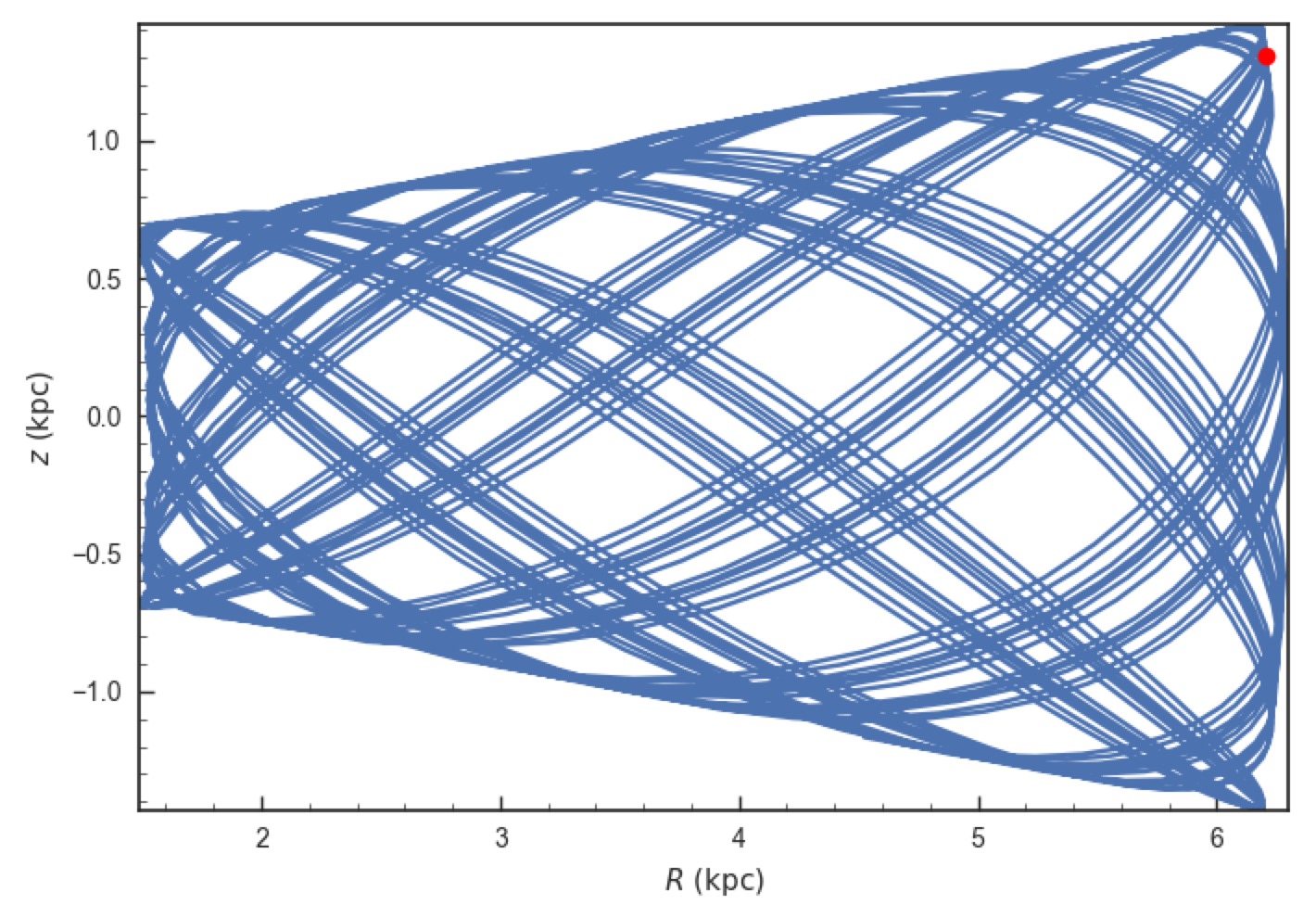We see that Omega Cen is currently close to its maximum distance from both the Galactic center and from the Galactic midplane (note that Omega Cen’s phase-space coordinates were updated internally in galpy after this plot was made and the orbit is now slightly different).

Similarly, you can do:

>>> o= Orbit.from_name('LMC')
>>> [o.ra(), o.dec(), o.dist(), o.pmra(), o.pmdec(), o.vlos()]
# [80.894200000000055, -69.756099999999847, 49.999999999999993, 1.909999999999999, 0.2290000000000037, 262.19999999999993]


It is also possible to initialize using multiple names, for example:

>>> o= Orbit.from_name(['LMC','SMC'])
>>> print(o.ra(),o.dec(),o.dist())
# [ 80.8942  13.1583] deg [-69.7561 -72.8003] deg [ 50.  60.] kpc


The names are stored in the name attribute:

>>> print(o.name)
# ['LMC', 'SMC']


The Orbit.from_name method attempts to resolve the name of the object in SIMBAD, and then use the observed coordinates found there to generate an Orbit instance. In order to query SIMBAD, Orbit.from_name requires the astroquery package to be installed. A small number of objects, mainly Milky Way globular clusters and dwarf satellite galaxies, have their phase-space coordinates stored in a file that is part of galpy and for these objects the values from this file are used rather than querying SIMBAD. Orbit.from_name supports tab completion in IPython/Jupyter for this list of objects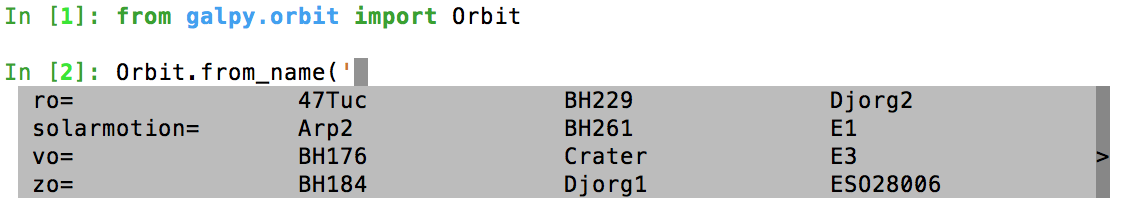The Orbit.from_name method also allows you to load some collections of objects in a simple manner. Currently, three collections are supported: ‘MW globular clusters’, ‘MW satellite galaxies’, and ‘solar system’. Specifying ‘MW globular clusters’ loads all of the Milky-Way globular clusters with data from Gaia DR2 (using the Vasiliev 2019 catalog):

>>> o= Orbit.from_name('MW globular clusters')
>>> print(len(o))
# 150
>>> print(o.name)
# ['NGC5286', 'Terzan12', 'Arp2', 'NGC5024', ... ]
>>> print(o.r())
# [  8.86999028   3.33270877  21.42173795  18.41411889, ...]


It is then easy to, for example, integrate the orbits of all Milky-Way globular clusters in MWPotential2014 and plot them in 3D:

>>> ts= numpy.linspace(0.,300.,1001)
>>> o.integrate(ts,MWPotential2014)
>>> o.plot3d(alpha=0.4)
>>> xlim(-100.,100.)
>>> ylim(-100.,100)
>>> gca().set_zlim3d(-100.,100);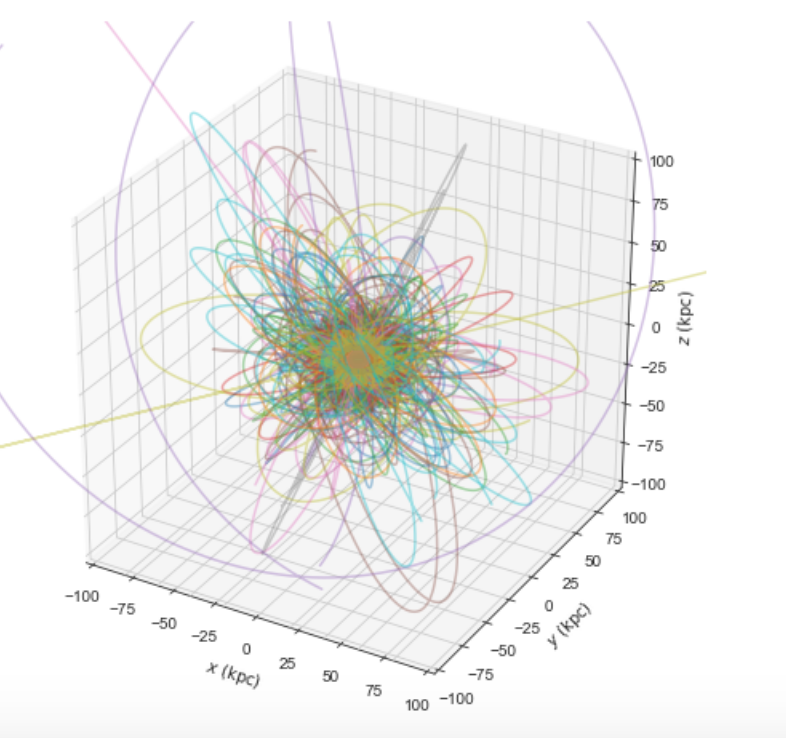Similarly, ‘MW satellite galaxies’ loads all of the Milky-Way satellite galaxies from Fritz et al. (2018):

>>> o= Orbit.from_name('MW satellite galaxies')
>>> print(len(o))
# 40
>>> print(o.name)
# ['AquariusII', 'BootesI', 'BootesII', 'CanesVenaticiI', 'CanesVenaticiII', 'Carina',
'CarinaII', 'CarinaIII', 'ComaBerenices', 'CraterII', 'Draco', 'DracoII', 'EridanusII',
'Fornax', 'GrusI', 'Hercules', 'HorologiumI', 'HydraII', 'HydrusI', 'LeoI', 'LeoII',
'LeoIV', 'LeoV', 'LMC', 'PhoenixI', 'PiscesII', 'ReticulumII', 'Sgr', 'Sculptor',
'Segue1', 'Segue2', 'SMC', 'Sextans', 'TriangulumII', 'TucanaII', 'TucanaIII',
'UrsaMajorI', 'UrsaMajorII', 'UrsaMinor', 'Willman1']
>>> print(o.r())
# [105.11517882  64.02897066  39.72074174 210.66938806 160.60529059
105.36807259  37.01725917  28.92738515  43.43084545 111.15279646
79.06498854  23.70062857 364.87901007 141.29780146 116.28700298
128.81345239  83.47228672 147.90512269  25.68800918 272.93146472
227.39435987 154.7574384  173.77516411  49.60813235 418.76813979
181.9540996   32.92030664  19.06561359  84.81046251  27.67609888
42.13122436  60.28760354  88.86197382  34.58139798  53.79147743
21.05413655 101.85224099  40.70060809  77.72601419  42.65853777] kpc


and we can integrate and plot them in 3D as above:

>>> o.plot3d(alpha=0.4)
>>> xlim(-400.,400.)
>>> ylim(-400.,400)
>>> gca().set_zlim3d(-400.,400)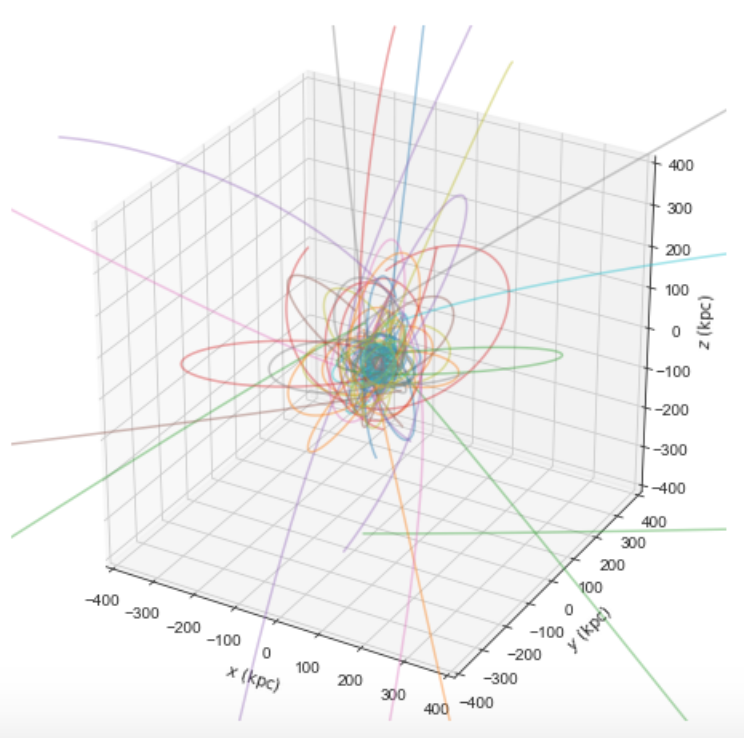Because MWPotential2014 has a relatively low-mass dark-matter halo, a bunch of the satellites are unbound (to make them bound, you can increase the mass of the halo by, for example, multiplying it by 1.5, as in MWPotential2014*= 1.5).

Finally, for illustrative purposes, the solar system is included as a collection as well. The solar system is set up such that the center of what is normally the Galactocentric coordinate frame in galpy is now the solar system barycenter and the coordinate frame is a heliocentric one. The solar system data are taken from Bovy et al. (2010) and they represent the positions and planets on April 1, 2009. To load the solar system do:

>>> o= Orbit.from_name('solar system')


Giving for example:

>>> print(o.name)
# ['Mercury', 'Venus', 'Earth', 'Mars', 'Jupiter', 'Saturn', 'Uranus', 'Neptune']


You can then, for example, integrate the solar system for 10 years as follows

>>> import astropy.units as u
>>> from galpy.potential import KeplerPotential
>>> from galpy.util.bovy_conversion import get_physical
>>> kp= KeplerPotential(amp=1.*u.Msun,**get_physical(o)) # Need to use **get_physical to get the ro= and vo= parameters, which differ from the default for the solar system
>>> ts= numpy.linspace(0.,10.,1001)*u.yr
>>> o.integrate(ts,kp)
>>> o.plot(d1='x',d2='y')


which givesNote that, as usual, physical outputs are in kpc, leading to very small numbers!

Tip

Setting up an Orbit instance without arguments will return an Orbit instance representing the Sun: o= Orbit(). This instance has physical units turned on by default, so methods will return outputs in physical units unless you o.turn_physical_off().

Warning

Orbits initialized using Orbit.from_name have physical output turned on by default, so methods will return outputs in physical units unless you o.turn_physical_off().

## Orbit integration¶

After an orbit is initialized, we can integrate it for a set of times ts, given as a numpy array. For example, in a simple logarithmic potential we can do the following

>>> from galpy.potential import LogarithmicHaloPotential
>>> lp= LogarithmicHaloPotential(normalize=1.)
>>> o= Orbit([1.,0.1,1.1,0.,0.1,0.])
>>> import numpy
>>> ts= numpy.linspace(0,100,10000)
>>> o.integrate(ts,lp)


to integrate the orbit from t=0 to t=100, saving the orbit at 10000 instances. In physical units, we can integrate for 10 Gyr as follows

>>> from astropy import units
>>> ts= numpy.linspace(0,10.,10000)*units.Gyr
>>> o.integrate(ts,lp)


Warning

When the integration times are not specified using a Quantity, they are assumed to be in natural units.

If we initialize the Orbit using a distance scale ro= and a velocity scale vo=, then Orbit plots and outputs will use physical coordinates (currently, times, positions, and velocities)

>>> op= Orbit([1.,0.1,1.1,0.,0.1,0.],ro=8.,vo=220.) #Use Vc=220 km/s at R= 8 kpc as the normalization
>>> op.integrate(ts,lp)


An Orbit instance containing multiple objects can be integrated in the same way and the orbit integration will be performed in parallel on machines with multiple cores. For the fast C integrators (see below), this parallelization is done using OpenMP in C and requires one to set the OMP_NUM_THREADS environment variable to control the number of cores used. The Python integrators are parallelized in Python and by default also use the OMP_NUM_THREADS variable to set the number of cores (but for the Python integrators this can be overwritten). A simple example is

>>> vxvvs= numpy.array([[1.,0.1,1.,0.1,-0.2,1.5],[0.1,0.3,1.1,-0.3,0.4,2.]])
>>> orbits= Orbit(vxvvs)
>>> orbits.integrate(ts,lp)
>>> print(orbits.R(ts).shape)
# (2,10000)
>>> print(orbits.R(ts))
# [[ 1.          1.00281576  1.00563403 ...,  1.05694767  1.05608923
#   1.0551804 ]
# [ 0.1         0.18647825  0.27361065 ...,  3.39447863  3.34992543
#   3.30527001]]


## Displaying the orbit¶

After integrating the orbit, it can be displayed by using the plot() function. The quantities that are plotted when plot() is called depend on the dimensionality of the orbit: in 3D the (R,z) projection of the orbit is shown; in 2D either (X,Y) is plotted if the azimuth is tracked and (R,vR) is shown otherwise; in 1D (x,vx) is shown. E.g., for the example given above,

>>> o.plot()


gives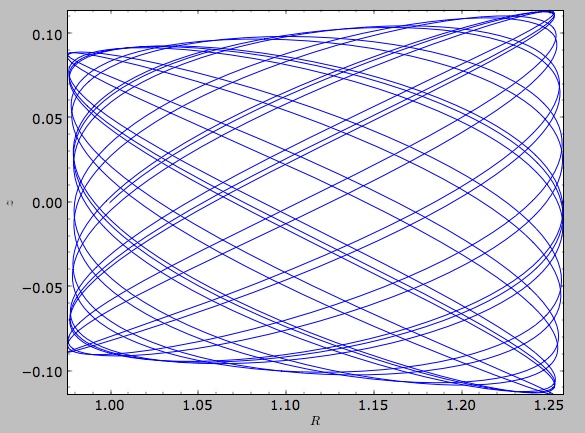If we do the same for the Orbit that has physical distance and velocity scales associated with it, we get the following

>>> op.plot()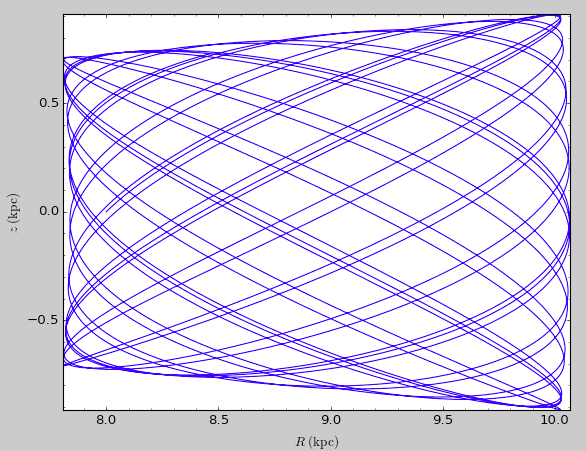If we call op.plot(use_physical=False), the quantities will be displayed in natural galpy coordinates.

Plotting an Orbit instance that consists of multiple objects plots all objects at once, e.g.,

>>> orbits.plot()


gives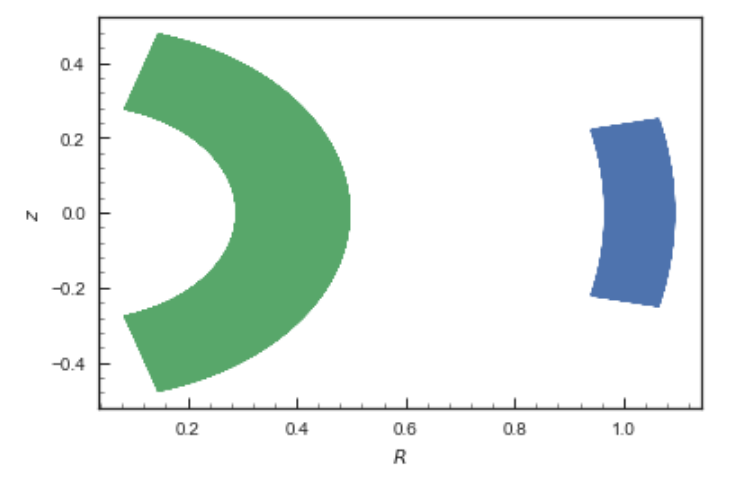Other projections of the orbit can be displayed by specifying the quantities to plot. E.g.,

>>> o.plot(d1='x',d2='y')


gives the projection onto the plane of the orbit: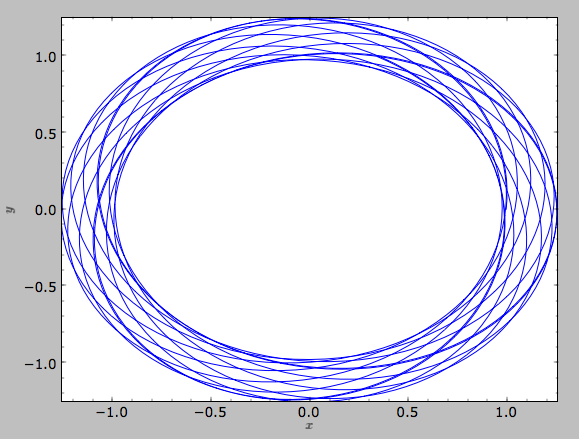while

>>> o.plot(d1='R',d2='vR')


gives the projection onto (R,vR):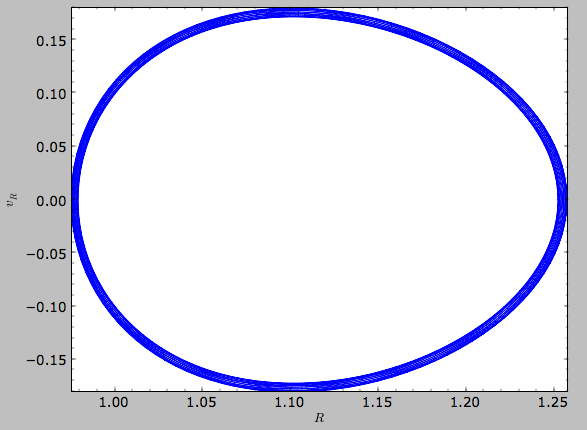We can also plot the orbit in other coordinate systems such as Galactic longitude and latitude

>>> o.plot('k.',d1='ll',d2='bb')


which shows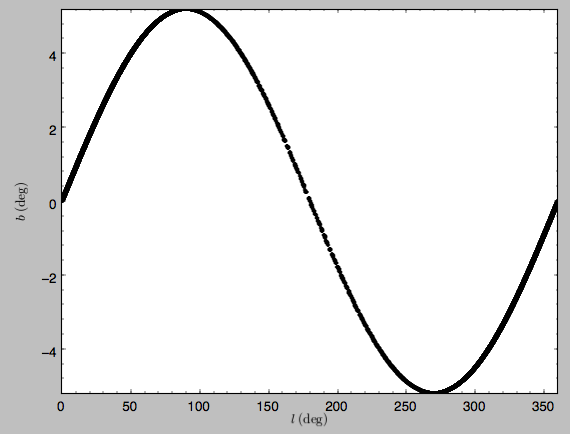or RA and Dec

>>> o.plot('k.',d1='ra',d2='dec')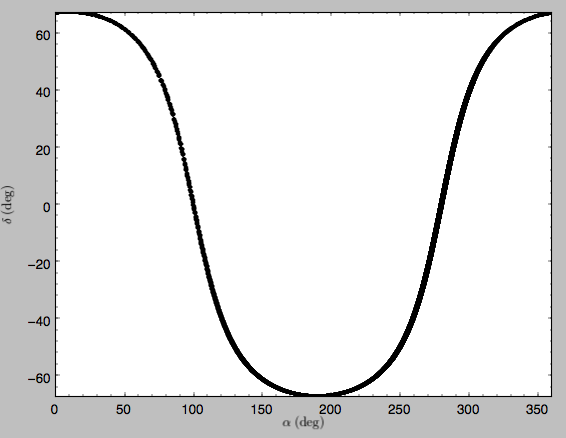See the documentation of the o.plot function and the o.ra(), o.ll(), etc. functions on how to provide the necessary parameters for the coordinate transformations.

It is also possible to plot quantities computed from the basic Orbit outputs like o.x(), o.r(), etc. For this to work, the numexpr module needs to be installed; this can be done using pip or conda. Then you can ask for plots like

>>> o.plot(d1='r',d2='vR*R/r+vz*z/r')


where d2= converts the velocity to spherical coordinates (this is currently not a pre-defined option). This gives the following orbit (which is closed in this projection, because we are using a spherical potential):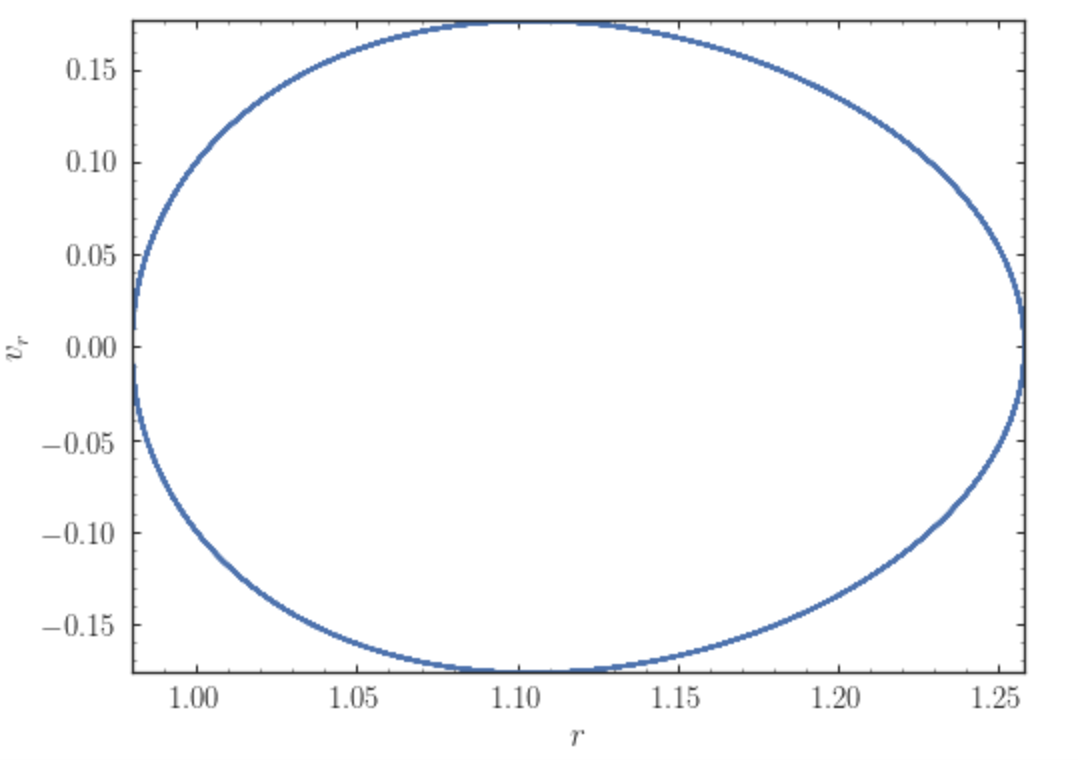You can also do more complex things like

>>> o.plot(d1='x',d2='y')
>>> o.plot(d1='R*cos(phi-{:f}*t)'.format(o.Op(quantity=False)),
d2='R*sin(phi-{:f}*t)'.format(o.Op(quantity=False)),
overplot=True)


which shows the orbit in the regular (x,y) frame as well as in a (x,y) frame that is rotating at the angular frequency of the orbit. When doing more complex calculations like this, you need to make sure that you are getting the units right: parameters param in the expression you provide are directly evaluated as o.param(), which depending on how you setup the object may or may not return output in physical units. The expression above is safe, because o.Op evaluated like this will be in a consistent unit system with the rest of the expression. Expressions cannot contain astropy Quantities (these cannot be parsed by the parser), which is why quantity=False is specified; this is also used internally.

Finally, it is also possible to plot arbitrary functions of time with Orbit.plot, by specifying d1= or d2= as a function. For example, to display the orbital velocity in the spherical radial direction, which we also did with the expression above, you can do the following

>>> o.plot(d1='r',
d2=lambda t: o.vR(t)*o.R(t)/o.r(t)+o.vz(t)*o.z(t)/o.r(t),
ylabel='v_r')


For a function like this, just specifying it as the expression d2='vR*R/r+vz*z/r' is much more convenient, but expressions that cannot be parsed automatically could be directly given as a function.

## Animating the orbit¶

Warning

Animating orbits is a new, experimental feature at this time that may be changed in later versions. It has only been tested in a limited fashion. If you are having problems with it, please open an Issue and list all relevant details about your setup (python version, jupyter version, browser, any error message in full). It may also be helpful to check the javascript console for any errors.

In a jupyter notebook or in jupyterlab (jupyterlab versions >= 0.33) you can also create an animation of an orbit after you have integrated it. For example, to do this for the op orbit from above (but only integrated for 2 Gyr to create a shorter animation as an example here), do

>>> op.animate()


This will create the following animation

Tip

There is currently no option to save the animation within galpy, but you could use screen capture software (for example, QuickTime’s Screen Recording feature) to record your screen while the animation is running and save it as a video.

animate has options to specify the width and height of the resulting animation, and it can also animate up to three projections of an orbit at the same time. For example, we can look at the orbit in both (x,y) and (R,z) at the same time with

>>> op.animate(d1=['x','R'],d2=['y','z'],width=800)


which gives

If you want to embed the animation in a webpage, you can obtain the necessary HTML using the _repr_html_() function of the IPython.core.display.HTML object returned by animate. By default, the HTML includes the entire orbit’s data, but animate also has an option to store the orbit in a separate JSON file that will then be loaded by the output HTML code.

animate also works in principle for Orbit instances containing multiple objects, but in practice the resulting animation is very slow once more than a few orbits/projections are used.

## Orbit characterization¶

The properties of the orbit can also be found using galpy. For example, we can calculate the peri- and apocenter radii of an orbit, its eccentricity, and the maximal height above the plane of the orbit

>>> o.rap(), o.rperi(), o.e(), o.zmax()
# (1.2581455175173673,0.97981663263371377,0.12436710999105324,0.11388132751079502)


or for multiple objects at once

>>> orbits.rap(), orbits.rperi(), orbits.e(), orbits.zmax()
# (array([ 1.0918143 ,  0.49557137]),
# array([ 0.96779816,  0.29150873]),
# array([ 0.06021334,  0.2592654 ]),
# array([ 0.24734084,  0.47327396]))


These four quantities can also be computed using analytical means (exact or approximations depending on the potential) by specifying analytic=True

>>> o.rap(analytic=True), o.rperi(analytic=True), o.e(analytic=True), o.zmax(analytic=True)
# (1.2581448917376636,0.97981640959995842,0.12436697719989584,0.11390708640305315)


or for multiple objects at once (this calculation is done in parallel on systems that support it)

>>> orbits.rap(analytic=True), orbits.rperi(analytic=True), orbits.e(analytic=True), orbits.zmax(analytic=True)
# (array([ 1.09181433,  0.49557137]),
# array([ 0.96779816,  0.29150873]),
# array([ 0.06021335,  0.2592654 ]),
# array([ 0.24734693,  0.4733304 ]))


We can also calculate the energy of the orbit, either in the potential that the orbit was integrated in, or in another potential:

>>> o.E(), o.E(pot=mp)
# (0.6150000000000001, -0.67390625000000015)


where mp is the Miyamoto-Nagai potential of Introduction: Rotation curves.

For the Orbit op that was initialized above with a distance scale ro= and a velocity scale vo=, these outputs are all in physical units

>>> op.rap(), op.rperi(), op.e(), op.zmax()
# (10.065158988860341,7.8385312810643057,0.12436696983841462,0.91105035688072711) #kpc
>>> op.E(), op.E(pot=mp)
# (29766.000000000004, -32617.062500000007) #(km/s)^2


We can also show the energy as a function of time (to check energy conservation)

>>> o.plotE(normed=True)


gives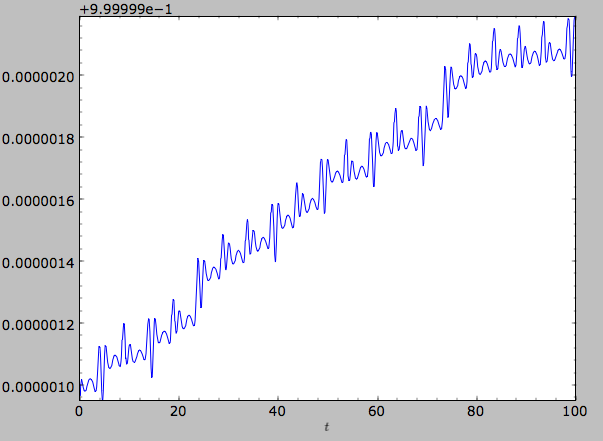We can specify another quantity to plot the energy against by specifying d1=. We can also show the vertical energy, for example, as a function of R

>>> o.plotEz(d1='R',normed=True)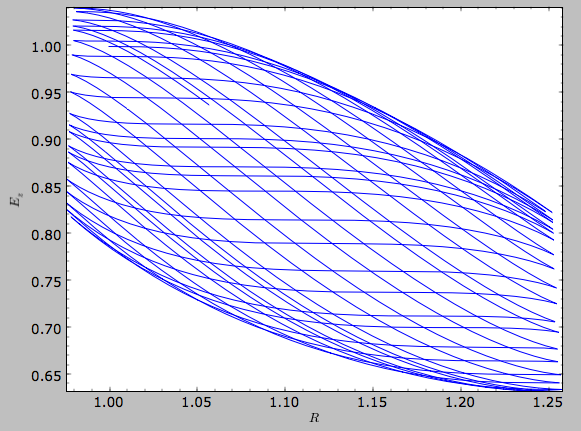## Fast orbit characterization¶

It is also possible to use galpy for the fast estimation of orbit parameters as demonstrated in Mackereth & Bovy (2018) via the Staeckel approximation (originally used by Binney (2012) for the appoximation of actions in axisymmetric potentials), without performing any orbit integration. The method uses the geometry of the orbit tori to estimate the orbit parameters. After initialising an Orbit instance, the method is applied by specifying analytic=True and selecting type='staeckel'.

>>> o.e(analytic=True, type='staeckel')


if running the above without integrating the orbit, the potential should also be specified in the usual way

>>> o.e(analytic=True, type='staeckel', pot=mp)


This interface automatically estimates the necessary delta parameter based on the initial condition of the Orbit object. (delta is the focal-length parameter of the prolate spheroidal coordinate system used in the approximation, see the documentation of the actionAngleStaeckel class).

While this is useful and fast for individual Orbit objects, it is likely that users will want to rapidly evaluate the orbit parameters of large numbers of objects. The easiest way to do this is by setting up an Orbit instance that contains all objects and call the same functions as above (in this case, the necessary delta parameter will be automatically determined for each object in the instance based on its initial condition)

>>> os= Orbit([R, vR, vT, z, vz, phi])
>>> os.e(analytic=True,type='staeckel',pot=mp)


In this case, the returned array has the same shape as the input R,vR,... arrays.

Rather than automatically estimating delta, you can specify an array for delta when calling os.e (or zmax, rperi, and rap), for example by first estimating good delta parameters as follows:

>>> from galpy.actionAngle import estimateDeltaStaeckel
>>> delta= estimateDeltaStaeckel(mp, R, z, no_median=True)


where no_median=True specifies that the function return the delta parameter at each given point rather than the median of the calculated deltas (which is the default option). Then one can compute the eccentricity etc. using individual delta values as:

>>> os.e(analytic=True,type='staeckel',pot=mp,delta=delta)


We can test the speed of this method in iPython by finding the parameters at 100000 steps along an orbit in MWPotential2014, like this

>>> o= Orbit([1.,0.1,1.1,0.,0.1,0.])
>>> ts = numpy.linspace(0,100,10000)
>>> o.integrate(ts,MWPotential2014)
>>> os= o(ts) # returns an Orbit instance with nt objects, each initialized at the position at one of the ts
>>> delta= estimateDeltaStaeckel(MWPotential2014,o.R(ts),o.z(ts),no_median=True)
>>> %timeit -n 10 os.e(analytic=True,pot=MWPotential2014,delta=delta)
# 584 ms ± 8.63 ms per loop (mean ± std. dev. of 7 runs, 1 loop each)


you can see that in this potential, each phase space point is calculated in roughly 60µs. further speed-ups can be gained by using the galpy.actionAngle.actionAngleStaeckelGrid module, which first calculates the parameters using a grid-based interpolation

>>> from galpy.actionAngle import actionAngleStaeckelGrid
>>> R, vR, vT, z, vz, phi = o.getOrbit().T
>>> aASG= actionAngleStaeckelGrid(pot=MWPotential2014,delta=0.4,nE=51,npsi=51,nLz=61,c=True,interpecc=True)
>>> %timeit -n 10 es, zms, rps, ras = aASG.EccZmaxRperiRap(R,vR,vT,z,vz,phi)
# 47.4 ms ± 5.11 ms per loop (mean ± std. dev. of 7 runs, 10 loops each)


where interpecc=True is required to perform the interpolation of the orbit parameter grid. Looking at how the eccentricity estimation varies along the orbit, and comparing to the calculation using the orbit integration, we see that the estimation good job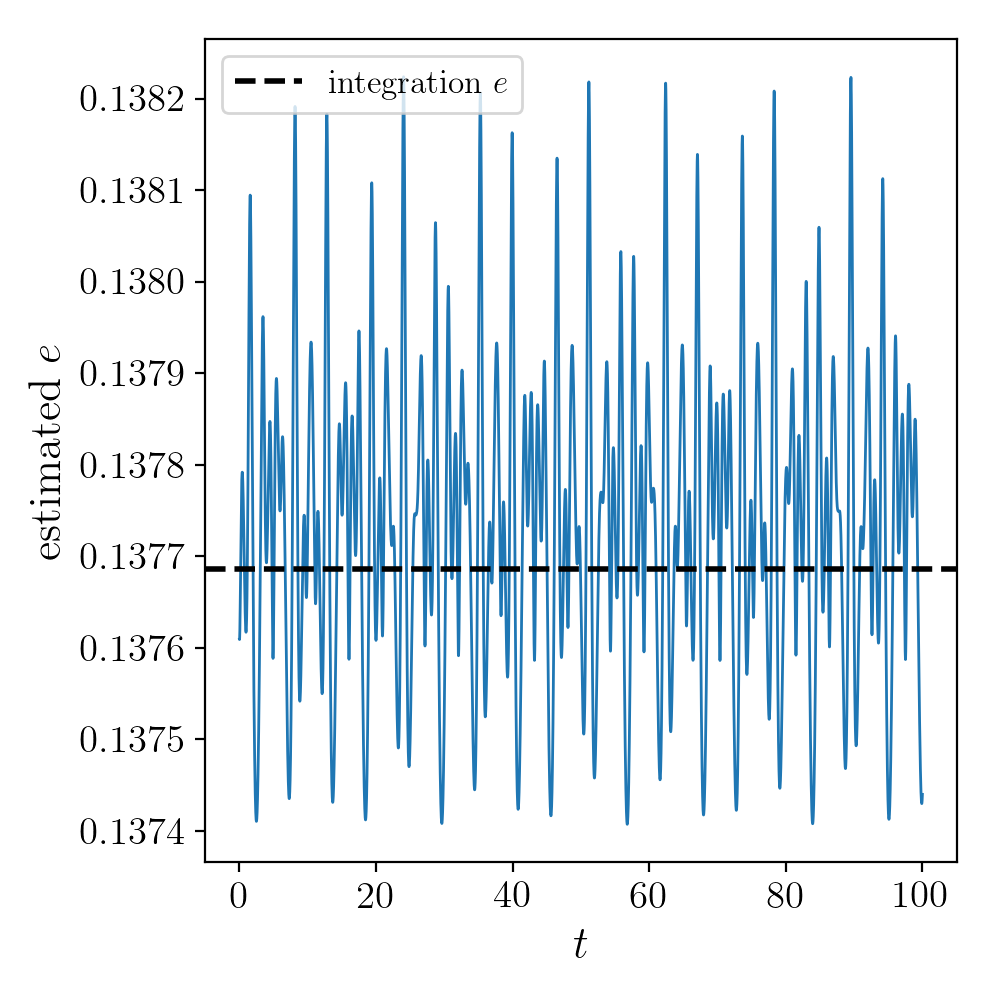## Accessing the raw orbit¶

The value of R, vR, vT, z, vz, x, vx, y, vy, phi, and vphi at any time can be obtained by calling the corresponding function with as argument the time (the same holds for other coordinates ra, dec, pmra, pmdec, vra, vdec, ll, bb, pmll, pmbb, vll, vbb, vlos, dist, helioX, helioY, helioZ, U, V, and W). If no time is given the initial condition is returned, and if a time is requested at which the orbit was not saved spline interpolation is used to return the value. Examples include

>>> o.R(1.)
# 1.1545076874679474
>>> o.phi(99.)
# 88.105603035901169
>>> o.ra(2.,obs=[8.,0.,0.],ro=8.)
# array([ 285.76403985])
>>> o.helioX(5.)
# array([ 1.24888927])
>>> o.pmll(10.,obs=[8.,0.,0.,0.,245.,0.],ro=8.,vo=230.)
# array([-6.45263888])


For the Orbit op that was initialized above with a distance scale ro= and a velocity scale vo=, the first of these would be

>>> op.R(1.)
# 9.2360614837829225 #kpc


which we can also access in natural coordinates as

>>> op.R(1.,use_physical=False)
# 1.1545076854728653


We can also specify a different distance or velocity scale on the fly, e.g.,

>>> op.R(1.,ro=4.) #different velocity scale would be vo=
# 4.6180307418914612


For Orbit instances that contain multiple objects, the functions above return arrays with the shape of the Orbit.

We can also initialize an Orbit instance using the phase-space position of another Orbit instance evaulated at time t. For example,

>>> newOrbit= o(10.)


will initialize a new Orbit instance with as initial condition the phase-space position of orbit o at time=10.. If multiple times are given, an Orbit instance with one object for each time will be instantiated (this works even if the original Orbit instance contained multiple objects already).

The whole orbit can also be obtained using the function getOrbit

>>> o.getOrbit()


which returns a matrix of phase-space points with dimensions [ntimes,nphasedim] or [shape,ntimes,nphasedim] for Orbit instances with multiple objects.

## Fast orbit integration and available integrators¶

The standard orbit integration is done purely in python using standard scipy integrators. When fast orbit integration is needed for batch integration of a large number of orbits, a set of orbit integration routines are written in C that can be accessed for most potentials, as long as they have C implementations, which can be checked by using the attribute hasC

>>> mp= MiyamotoNagaiPotential(a=0.5,b=0.0375,amp=1.,normalize=1.)
>>> mp.hasC
# True


Fast C integrators can be accessed through the method= keyword of the orbit.integrate method. Currently available integrators are

• rk4_c
• rk6_c
• dopr54_c
• dop853_c

which are Runge-Kutta and Dormand-Prince methods. There are also a number of symplectic integrators available

• leapfrog_c
• symplec4_c
• symplec6_c

The higher order symplectic integrators are described in Yoshida (1993). In pure Python, the available integrators are

• leapfrog
• odeint
• dop853

For most applications I recommend symplec4_c or dop853_c, which are speedy and reliable. For example, compare

>>> o= Orbit([1.,0.1,1.1,0.,0.1])
>>> timeit(o.integrate(ts,mp,method='leapfrog'))
# 1.34 s ± 41.8 ms per loop (mean ± std. dev. of 7 runs, 1 loop each)
>>> timeit(o.integrate(ts,mp,method='leapfrog_c'))
# galpyWarning: Using C implementation to integrate orbits
# 91 ms ± 2.42 ms per loop (mean ± std. dev. of 7 runs, 10 loops each)
>>> timeit(o.integrate(ts,mp,method='symplec4_c'))
# galpyWarning: Using C implementation to integrate orbits
# 9.67 ms ± 48.3 µs per loop (mean ± std. dev. of 7 runs, 100 loops each)
>>> timeit(o.integrate(ts,mp,method='dop853_c'))
# 4.65 ms ± 86.8 µs per loop (mean ± std. dev. of 7 runs, 100 loops each)


If the C extensions are unavailable for some reason, I recommend using the odeint pure-Python integrator, as it is the fastest. Using the same example as above

>>> o= Orbit([1.,0.1,1.1,0.,0.1])
>>> timeit(o.integrate(ts,mp,method='leapfrog'))
# 2.62 s ± 128 ms per loop (mean ± std. dev. of 7 runs, 1 loop each)
>>> timeit(o.integrate(ts,mp,method='odeint'))
# 153 ms ± 2.59 ms per loop (mean ± std. dev. of 7 runs, 10 loops each)
>>> timeit(o.integrate(ts,mp,method='dop853'))
# 1.61 s ± 218 ms per loop (mean ± std. dev. of 7 runs, 1 loop each)


## Integration of the phase-space volume¶

galpy further supports the integration of the phase-space volume through the method integrate_dxdv, although this is currently only implemented for two-dimensional orbits (planarOrbit). As an example, we can check Liouville’s theorem explicitly. We initialize the orbit

>>> o= Orbit([1.,0.1,1.1,0.])


and then integrate small deviations in each of the four phase-space directions

>>> ts= numpy.linspace(0.,28.,1001) #~1 Gyr at the Solar circle
>>> o.integrate_dxdv([1.,0.,0.,0.],ts,mp,method='dopr54_c',rectIn=True,rectOut=True)
>>> dx= o.getOrbit_dxdv()[-1,:] # evolution of dxdv along the orbit
>>> o.integrate_dxdv([0.,1.,0.,0.],ts,mp,method='dopr54_c',rectIn=True,rectOut=True)
>>> dy= o.getOrbit_dxdv()[-1,:]
>>> o.integrate_dxdv([0.,0.,1.,0.],ts,mp,method='dopr54_c',rectIn=True,rectOut=True)
>>> dvx= o.getOrbit_dxdv()[-1,:]
>>> o.integrate_dxdv([0.,0.,0.,1.],ts,mp,method='dopr54_c',rectIn=True,rectOut=True)
>>> dvy= o.getOrbit_dxdv()[-1,:]


We can then compute the determinant of the Jacobian of the mapping defined by the orbit integration from time zero to the final time

>>> tjac= numpy.linalg.det(numpy.array([dx,dy,dvx,dvy]))


This determinant should be equal to one

>>> print(tjac)
# 0.999999991189
>>> numpy.fabs(tjac-1.) < 10.**-8.
# True


The calls to integrate_dxdv above set the keywords rectIn= and rectOut= to True, as the default input and output uses phase-space volumes defined as (dR,dvR,dvT,dphi) in cylindrical coordinates. When rectIn or rectOut is set, the in- or output is in rectangular coordinates ([x,y,vx,vy] in two dimensions).

Implementing the phase-space integration for three-dimensional FullOrbit instances is straightforward and is part of the longer term development plan for galpy. Let the main developer know if you would like this functionality, or better yet, implement it yourself in a fork of the code and send a pull request!

## Example: The eccentricity distribution of the Milky Way’s thick disk¶

A straightforward application of galpy’s orbit initialization and integration capabilities is to derive the eccentricity distribution of a set of thick disk stars. We start by downloading the sample of SDSS SEGUE (2009AJ….137.4377Y) thick disk stars compiled by Dierickx et al. (2010arXiv1009.1616D) from CDS at this link. Downloading the table and the ReadMe will allow you to read in the data using astropy.io.ascii like so

>>> from astropy.io import ascii
>>> vxvv = numpy.dstack([dierickx['RAdeg'], dierickx['DEdeg'], dierickx['Dist']/1e3, dierickx['pmRA'], dierickx['pmDE'], dierickx['HRV']])


After reading in the data (RA,Dec,distance,pmRA,pmDec,vlos; see above) as a vector vxvv with dimensions [6,ndata] we (a) define the potential in which we want to integrate the orbits, and (b) integrate all orbits and compute their eccentricity numerically from the orbit integration and analytically following the Staeckel approximation method (the following takes lots of memory; you might want to slice the orbits object to a smaller number to run this code faster)

>>> ts= np.linspace(0.,20.,10000)
>>> lp= LogarithmicHaloPotential(normalize=1.)
>>> e_ana= orbits.e(analytic=True,pot=lp,delta=1e-6)
>>> orbits.integrate(ts,lp)
>>> e_int= orbits.e()


We then find the following eccentricity distribution (from the numerical eccentricities)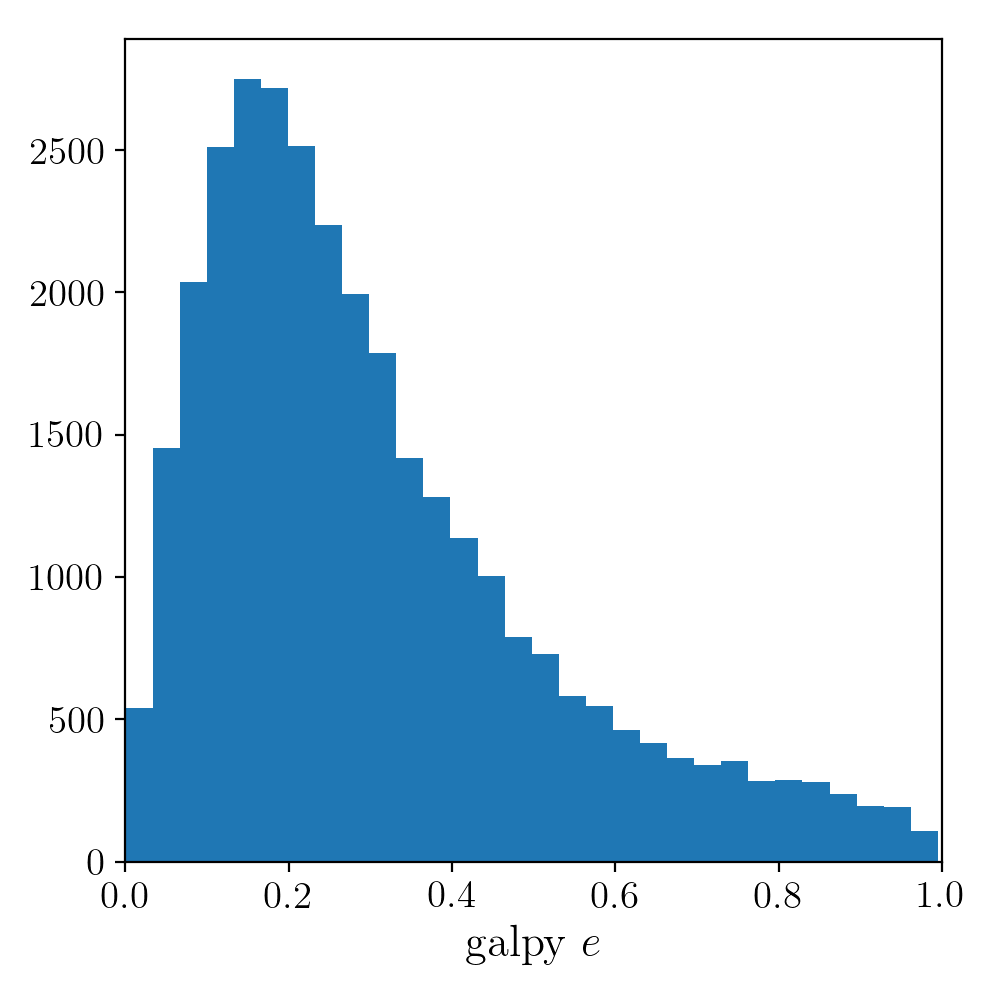The eccentricity calculated by integration in galpy compare well with those calculated by Dierickx et al., except for a few objects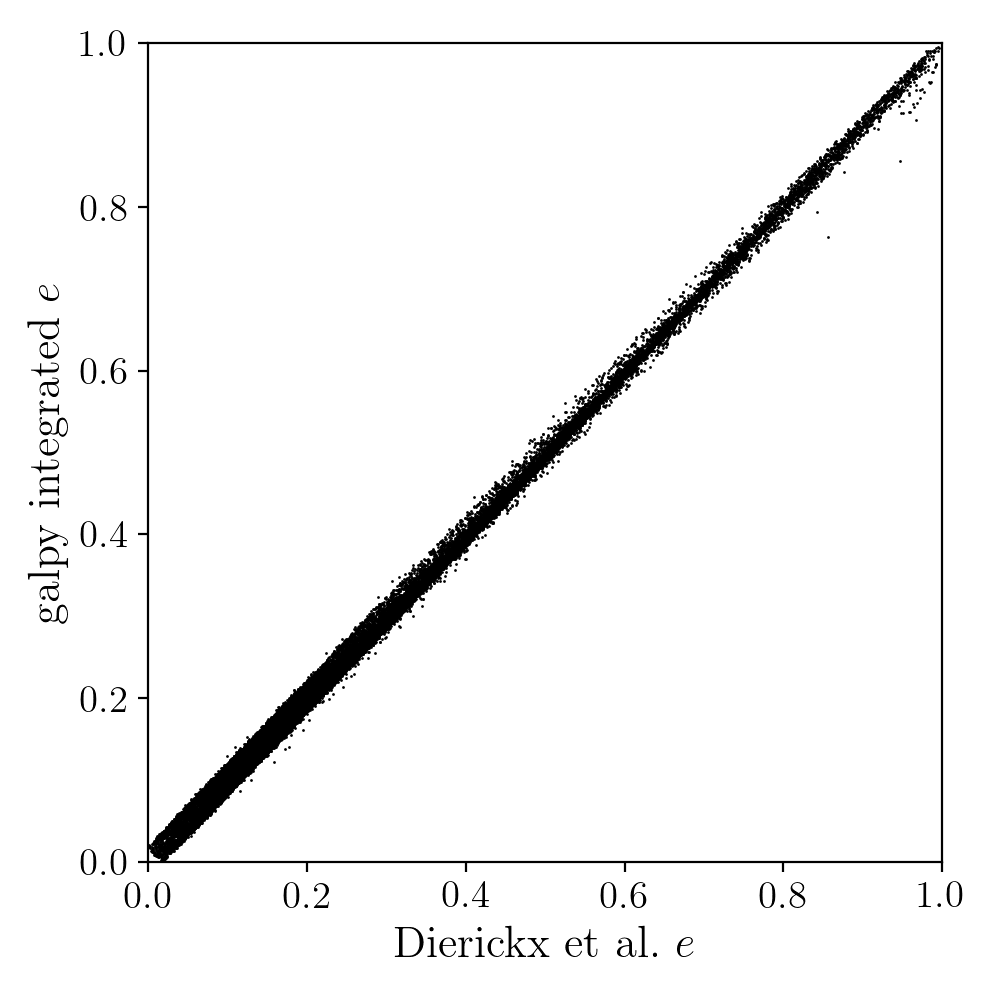and the analytical estimates are equally as good: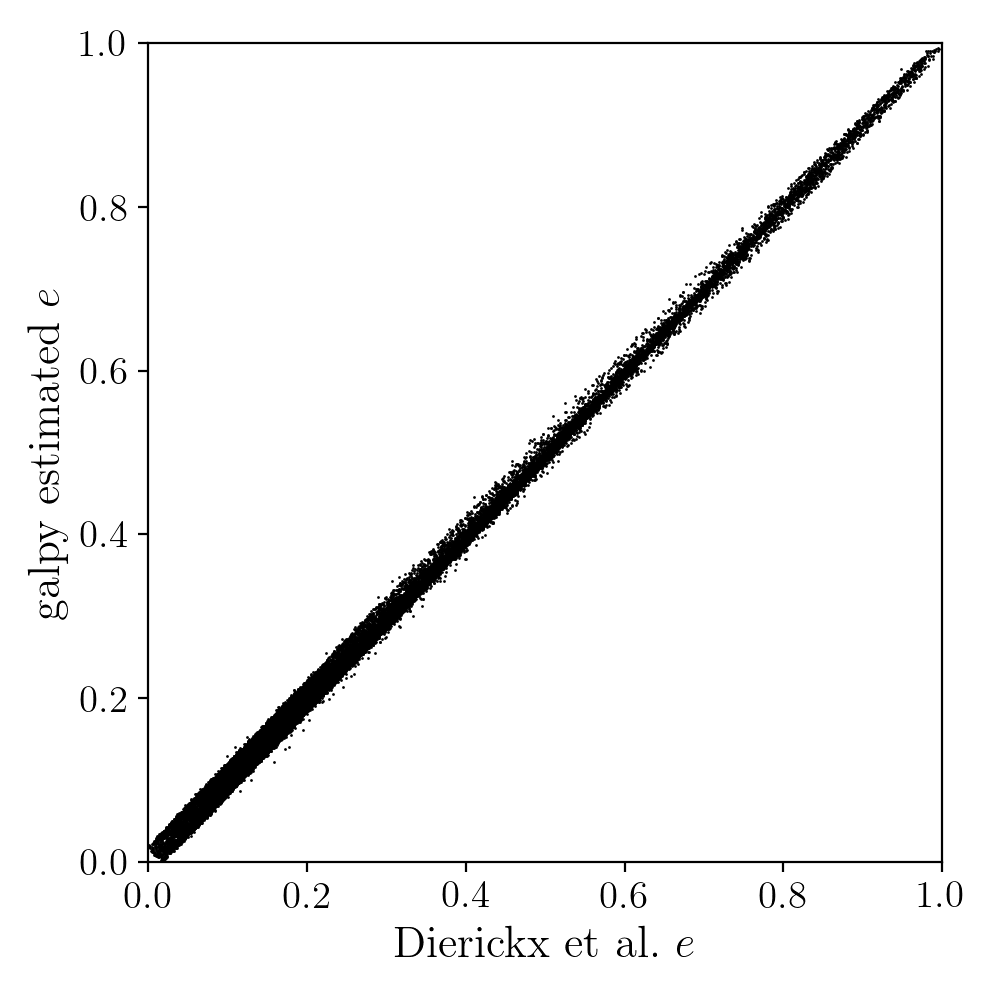In comparing the analytic and integrated eccentricity estimates - one can see that in this case the estimation is almost exact, due to the spherical symmetry of the chosen potential: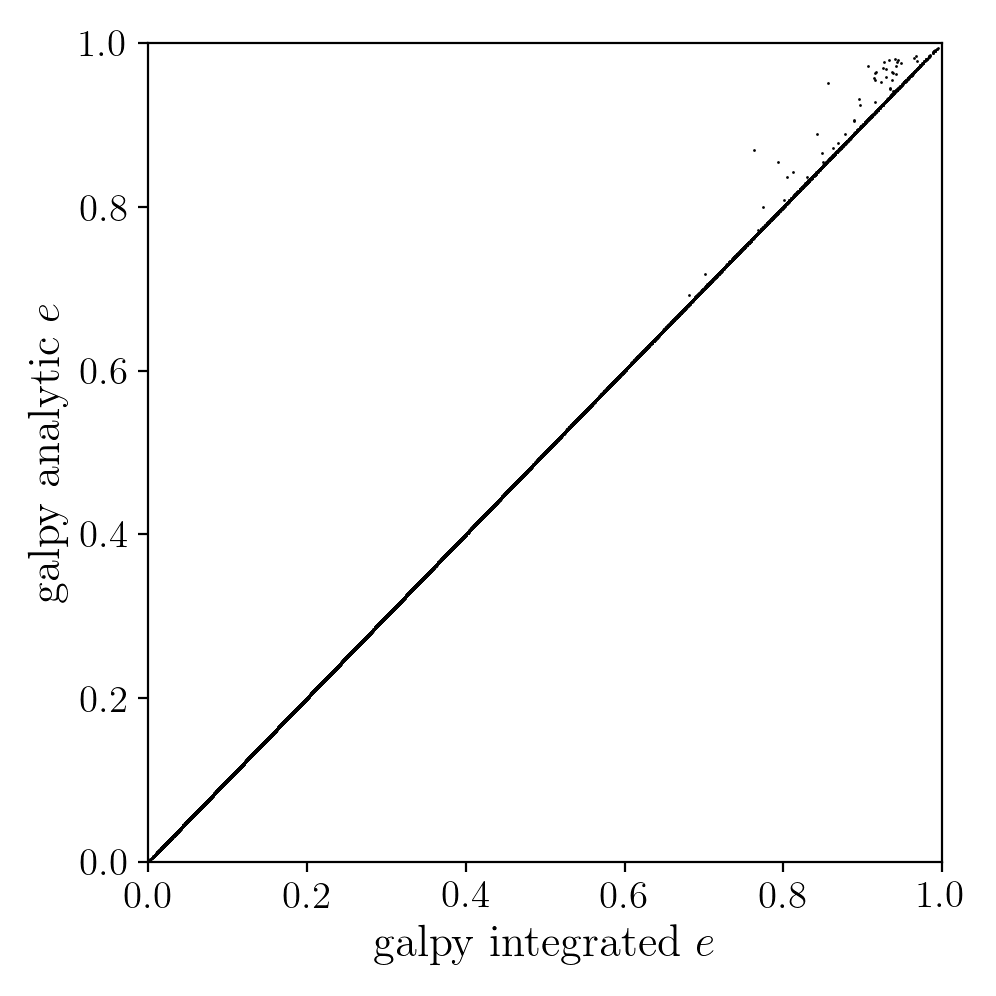A script that calculates and plots everything can be downloaded here. To generate the plots just run:

python dierickx_eccentricities.py ../path/to/folder


specifiying the location you want to put the plots and data.

Alternatively - one can transform the observed coordinates into spherical coordinates and perform the estimations in one batch using the actionAngle interface, which takes considerably less time:

>>> from galpy import actionAngle
>>> deltas = actionAngle.estimateDeltaStaeckel(lp, Rphiz[:,0], Rphiz[:,2], no_median=True)
>>> aAS = actionAngleStaeckel(pot=lp, delta=0.)
>>> par = aAS.EccZmaxRperiRap(Rphiz[:,0], vRvTvz[:,0], vRvTvz[:,1], Rphiz[:,2], vRvTvz[:,2], Rphiz[:,1], delta=deltas)


The above code calculates the parameters in roughly 100ms on a single core.

## Example: The orbit of the Large Magellanic Cloud in the presence of dynamical friction¶

As a further example of what you can do with galpy, we investigate the Large Magellanic Cloud’s (LMC) past and future orbit. Because the LMC is a massive satellite of the Milky Way, its orbit is affected by dynamical friction, a frictional force of gravitational origin that occurs when a massive object travels through a sea of low-mass objects (halo stars and dark matter in this case). First we import all the necessary packages:

>>> from astropy import units
>>> from galpy.potential import MWPotential2014, ChandrasekharDynamicalFrictionForce
>>> from galpy.orbit import Orbit


(also do %pylab inline if running this in a jupyter notebook or turn on the pylab option in ipython for plotting). We can load the current phase-space coordinates for the LMC using the Orbit.from_name function described above:

>>> o= Orbit.from_name('LMC')


We will use MWPotential2014 as our Milky-Way potential model. Because the LMC is in fact unbound in MWPotential2014, we increase the halo mass by 50% to make it bound (this corresponds to a Milky-Way halo mass of $$\approx 1.2\,\times 10^{12}\,M_\odot$$, a not unreasonable value). We can adjust a galpy Potential’s amplitude simply by multiplying the potential by a number, so to increase the mass by 50% we do

>>> MWPotential2014*= 1.5


Let us now integrate the orbit backwards in time for 10 Gyr and plot it:

>>> ts= numpy.linspace(0.,-10.,1001)*units.Gyr
>>> o.integrate(ts,MWPotential2014)
>>> o.plot(d1='t',d2='r')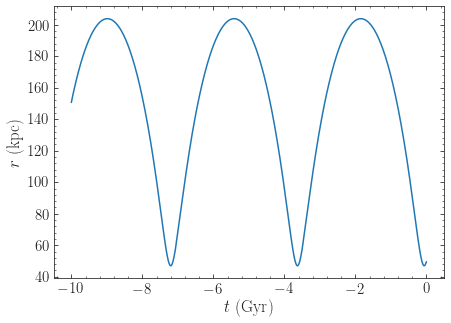We see that the LMC is indeed bound, with an apocenter just over 250 kpc. Now let’s add dynamical friction for the LMC, assuming that its mass is $$5\times 10^{10}\,M_\odot$$. We setup the dynamical-friction object:

>>> cdf= ChandrasekharDynamicalFrictionForce(GMs=5.*10.**10.*units.Msun,rhm=5.*units.kpc,
dens=MWPotential2014)


Dynamical friction depends on the velocity distribution of the halo, which is assumed to be an isotropic Gaussian distribution with a radially-dependent velocity dispersion. If the velocity dispersion is not given (like in the example above), it is computed from the spherical Jeans equation. We have set the half-mass radius to 5 kpc for definiteness. We now make a copy of the orbit instance above and integrate it in the potential that includes dynamical friction:

>>> odf= o()
>>> odf.integrate(ts,MWPotential2014+cdf)


Overlaying the orbits, we can see the difference in the evolution:

>>> o.plot(d1='t',d2='r',label=r'$\mathrm{No\ DF}$')
>>> odf.plot(d1='t',d2='r',overplot=True,label=r'$\mathrm{DF}, M=5\times10^{10}\,M_\odot$')
>>> ylim(0.,400.)
>>> legend()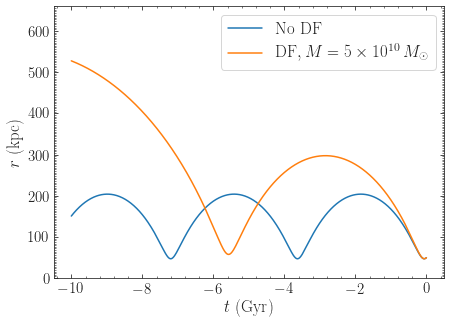We see that dynamical friction removes energy from the LMC’s orbit, such that its past apocenter is now around 400 kpc rather than 250 kpc! The period of the orbit is therefore also much longer. Clearly, dynamical friction has a big impact on the orbit of the LMC.

Recent observations have suggested that the LMC may be even more massive than what we have assumed so far, with masses over $$10^{11}\,M_\odot$$ seeming in good agreement with various observations. Let’s see how a mass of $$10^{11}\,M_\odot$$ changes the past orbit of the LMC. We can change the mass of the LMC used in the dynamical-friction calculation as

>>> cdf.GMs= 10.**11.*units.Msun


This way of changing the mass is preferred over re-initializing the ChandrasekharDynamicalFrictionForce object, because it avoids having to solve the Jeans equation again to obtain the velocity dispersion. Then we integrate the orbit and overplot it on the previous results:

>>> odf2= o()
>>> odf2.integrate(ts,MWPotential2014+cdf)


and

>>> o.plot(d1='t',d2='r',label=r'$\mathrm{No\ DF}$')
>>> odf.plot(d1='t',d2='r',overplot=True,label=r'$\mathrm{DF}, M=5\times10^{10}\,M_\odot$')
>>> odf2.plot(d1='t',d2='r',overplot=True,label=r'$\mathrm{DF}, M=1\times10^{11}\,M_\odot$')
>>> ylim(0.,740.)
>>> legend()


which gives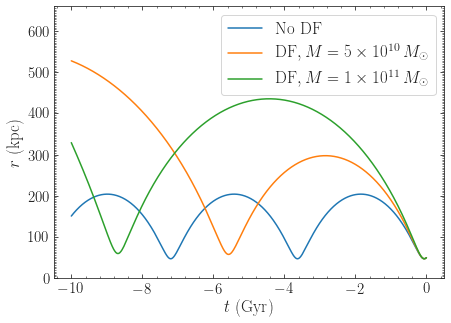Now the apocenter increases to about 600 kpc and the LMC doesn’t perform a full orbit over the last 10 Gyr.

Finally, let’s see what will happen in the future if the LMC is as massive as $$10^{11}\,M_\odot$$. We simply flip the sign of the integration times to get the future trajectory:

>>> odf2.integrate(-ts[-ts < 9*units.Gyr],MWPotential2014+cdf)
>>> odf2.plot(d1='t',d2='r')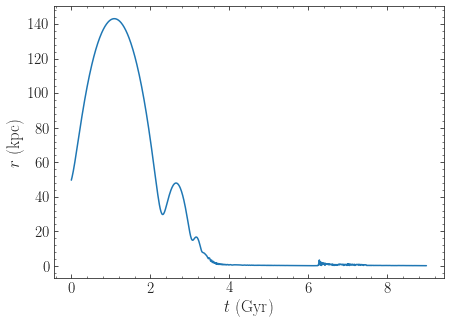Because of the large effect of dynamical friction, the LMC will merge with the Milky-Way in about 4 Gyr after a few more pericenter passages. Note that we have not taken any mass-loss into account. Because mass-loss would lead to a smaller dynamical-friction force, this would somewhat increase the merging timescale, but dynamical friction will inevitably lead to the merger of the LMC with the Milky Way.

Warning

When using dynamical friction, if the radius gets very small, the integration sometimes becomes very erroneous, which can lead to a big, unphysical kick (even though we turn off friction at very small radii); this is the reason why we have limited the future integration to 9 Gyr in the example above. When using dynamical friction, inspect the full orbit to make sure to catch whether a merger has happened.